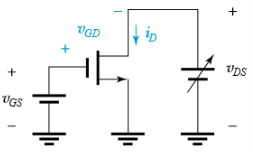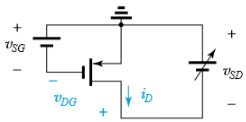Courses

# Test: MOSFETs Current Voltage Characterisitcs

## 10 Questions MCQ Test Electronic Devices | Test: MOSFETs Current Voltage Characterisitcs

Description
This mock test of Test: MOSFETs Current Voltage Characterisitcs for Electrical Engineering (EE) helps you for every Electrical Engineering (EE) entrance exam. This contains 10 Multiple Choice Questions for Electrical Engineering (EE) Test: MOSFETs Current Voltage Characterisitcs (mcq) to study with solutions a complete question bank. The solved questions answers in this Test: MOSFETs Current Voltage Characterisitcs quiz give you a good mix of easy questions and tough questions. Electrical Engineering (EE) students definitely take this Test: MOSFETs Current Voltage Characterisitcs exercise for a better result in the exam. You can find other Test: MOSFETs Current Voltage Characterisitcs extra questions, long questions & short questions for Electrical Engineering (EE) on EduRev as well by searching above.
QUESTION: 1

### If a MOSFET is to be used in the making of an amplifier then it must work in

Solution:

Only in the saturation region a MOSFET can operate as an amplifier.

QUESTION: 2

### For MOSFET is to be used as a switch then it must operate in

Solution:

In both regions it can perform the task of a switch.

QUESTION: 3

### (Q.3 & Q.4) Using the circuit shown below,Q. Determine the conditions in which the MOSFET is operating in the triode region. i. VGD > Vt (Threshold voltage) ii. VDS > VOV iii. ID ∝ (VOV – 0.5VDS)VDS

Solution:

Only the points I and iii are correct and ii is false.

QUESTION: 4

Determine the conditions in which the MOSFET is operating in the saturation region
i. VGD > Vt (Threshold voltage)
ii. VDS > VOV
iii. ID ∝ (VOV)2

Solution:

i is false and ii and iii are true.

QUESTION: 5

In the saturation region of the MOSFET the saturation current is

Solution:

Saturation current does not depends on the voltage difference between the source and the drain in the saturation region of a MOSFET.

QUESTION: 6

An n-channel MOSFET operating with VOV=0.5V exhibits a linear resistance = 1 kΩ when VDS is very small. What is the value of the device transconductance parameter kn?

Solution:

Use the standard mathematical expression to determine the value of kn.

QUESTION: 7

An NMOS transistor is operating at the edge of saturation with an overdrive voltage VOV and a drain current ID. If is VOV is doubled, and we must maintain operation at the edge of saturation, what value of drain current results?

Solution:

I0 is directly proportional to VOS.

QUESTION: 8

(Q.8-Q.10) Using the circuit below answer the questionQ. Which of the following is true for the triode region?

Solution:

VDG > |Vtp| and VSD < |VOV|.

QUESTION: 9

Which of the following is true for the saturation region?

Solution:

It is a characteristic for the saturation region.

QUESTION: 10

The current iD

Solution:

Use the standard mathematical expressions for i0 in different regions.